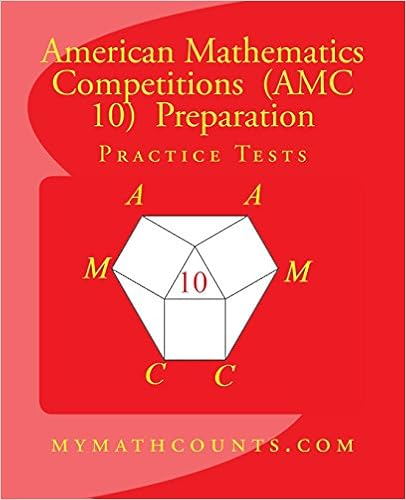HomeAMC 10 Preparation Books (hard copy)

# Buy all six volumes: \$106.67## Click to order.

http://mymathcounts.com/new/books/amc10/hard_copy.php

This book can be used by students preparing for AMC 10.  Each chapter consists of  (1) basic skill and knowledge section with plenty of examples, (2) about 30 exercise problems, and (3) detailed solutions to all problems.

##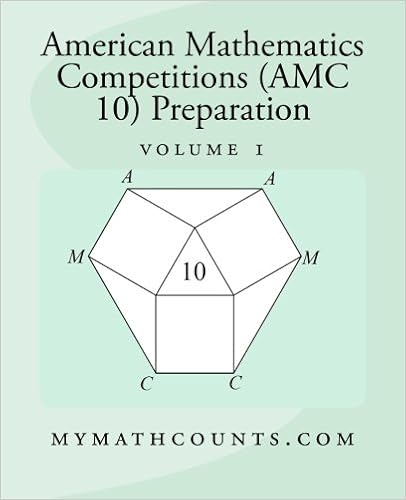Chapter 1.  Logic Reasoning                                                                          1

Chapter 2. Algebraic Simplification                                                               30

Chapter 3. Percentages                                                                                   53

Chapter 4. Ratios and Proportions                                                                  74

Chapter 5. Factoring   Methods                                                                      99

Chapter 6. Pythagorean Theorem                                                                   126

Index                                                                                                               163

##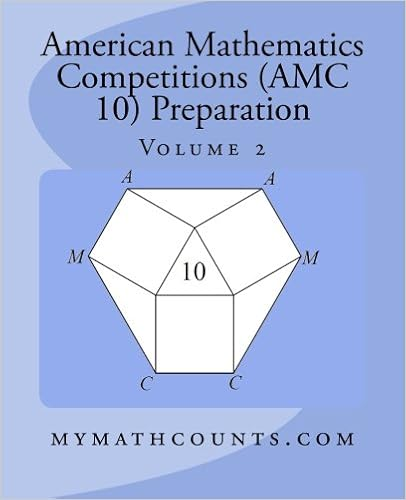Chapter 7. Triangle Area and Area Method                                                   1

Chapter 8. Character of Digits                                                                        36

Chapter 9. Exponents                                                                                     61

Chapter 10. Word Problem – Distance and Speed                                         86

Chapter 11. Divisibility                                                                                   111

Chapter 12. Similar Triangles                                                                          145

Index                                                                                                               178

# Volume 3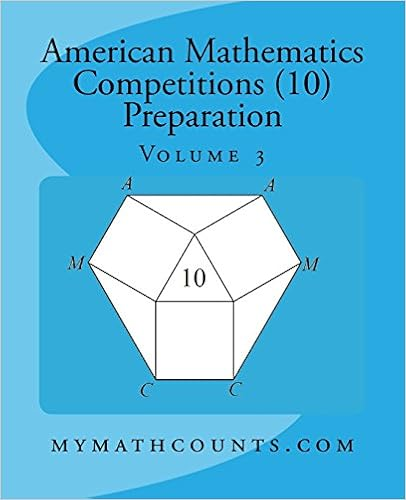Chapter 13. Prime Numbers                                                                            1

Chapter 15. Arithmetic and Geometric Sequences                                         60

Chapter 16. Circle – Radius, Circumference, and Areas                                93

Chapter 17. Coordinate Geometry – Lines and Circles                                  131

Chapter 18. Mean, Median, Mode, and Range                                              171

Index                                                                                                               198

# Volume 4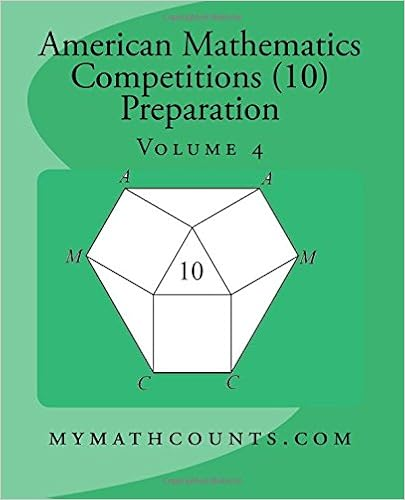Chapter 19. Counting by Permutation and Combination                               1

Chapter 20. Geometry – the Mass Point Method                                           33

Chapter 21. Counting  – Balls and Boxes                                                      70

Chapter 22. Geometry – Angle Bisector and Median                                                99

Chapter 23. Counting with Geometric Figures                                               131

Index                                                                                                               169

# Volume 5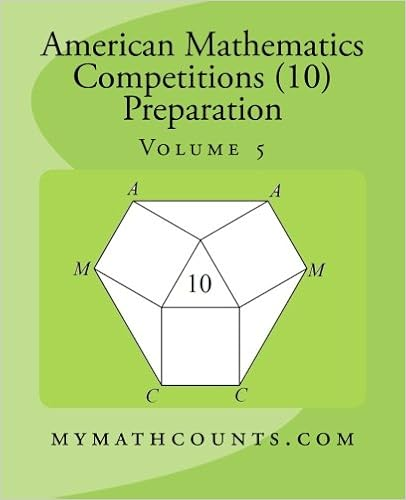Chapter 24. Factors and Divisors                                                                   1

Chapter 25. Equations Solving Skills                                                             30

Chapter 26.  Solving System of Equations                                                     58

Chapter 27. Sum and Products of Roots                                                        86

Chapter 28. Solid Geometry                                                                           120

Index                                                                                                               155
Volume 6 10 Practice Tests with detailed solutions)